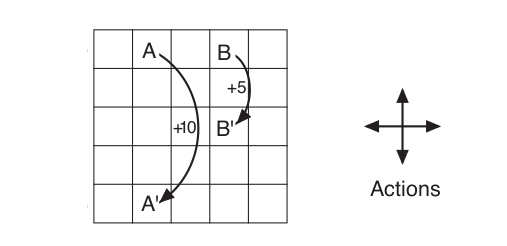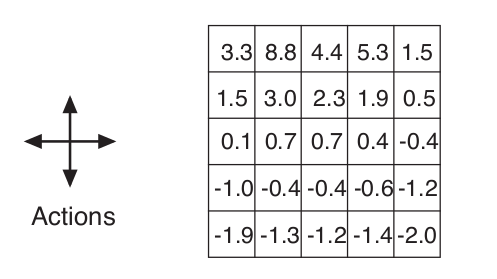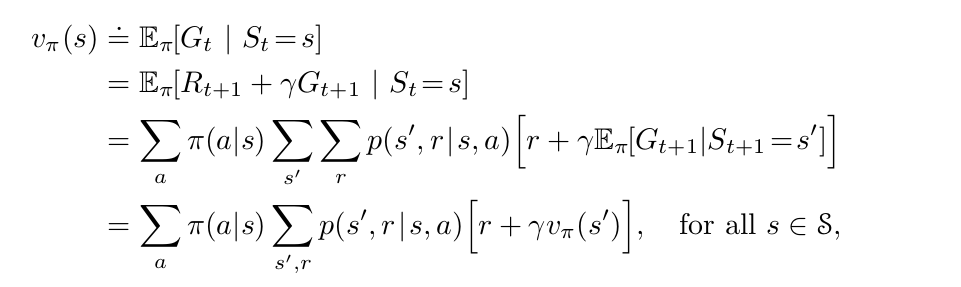## Coding the GridWorld Example from DeepMind’s Reinforcement Learning Course in Python

Here I calculate the state value functions for all states in the GridWorld example from the well renowned David Silver’s Reinforcement Learning Course.Fig 3.2Fig 3.3 

Suppose the policy is that the agent selects all four actions with equal probability in all four states. Here in Fig 3.3 the same grid is shown with the State Value Functions for this policy for all states calculated using the following formula (for the discounted reward case equal to 0.9)Formula 3.14 

If the various tough words like states, state value functions, MDP, reward, policy etc. confuse you , believe me they are much easier than it usually sounds. To learn more about them you should go through David Silver’s Reinforcement Learning Course  or the book “Reinforcement Learning: Second Edition” by Richard S. Sutton and Andrew G. Barto .

So this was all that was given in the example. But I was pretty curious about the real mathematics of how the state value functions of the gridworld were calculated. So I decided to write a python program to calculate them and see if I can get the same values. So lets see my code and how I worked through the problem. ( You can see the full code in my github repo here https://github.com/realdiganta/gridworld)

Now the first thing to do is make a grid. I used numpy to make a (5,5) grid with all values initialized to zero.

Okay so now if we see there are 4 different types of states:-

1. The state A, moving out from which yields us +10 reward and then moves to cell A’ (no matter if we go up, down, left or right)
2. State B, moving out from which yields us +5 reward and moves to cell B’ (no matter if we go up, down, left or right)
3. States from which we may go out of the grid. So suppose we take the cell (state) in the first row, first column. If we go UP from this state then we go out of the grid. This will yield us a reward of -1 and we will come back to the state from where we started.
4. Other than that moving out of any other cell(state) yields us a reward of 0 and we move to the new cell.

So our first step is to represent the value functions for a particular state in the grid which we can easily do by indexing that particular state/cell. And we can represent going left, right , up , down by simply adding or subtracting 1 from the index as required. However if going in any direction moves us out of the grid, we will initialize the value of that cell to be None, else it will be the value of the new cell where we end up.

Our goal is to calculate the State Value Functions of each of these states where the policy is that there is a equal probability of moving in any of the 4 directions. So now if you remember, the state value function of a particular state is the immediate reward we get plus the value function of the state where we end for a particular action as per our policy.

For example:- for the first cell in the first row, first column here is the calculation — →

1. If we go up — — -> 0.25 * (-1 + 0.9 * 0) = -0.25# Let me explain. since there is a equal probability of going in any of the directions so the probability of going up is 0.25 then -1 is the immediate reward we get since going up means we are moving out of the grid. Since, moving out of the grid lands us in the same state, so 0 is the value function of the current state. And 0.9 is the discounted reward case.
2. Similarly if we go left — — -> 0.25*(-1 + 0.9*0) = -0.25
3. If we go right — — -> 0.25 *(0 + 0.9*0) = 0 # Here there are only two differences. First the immediate reward is 0, because we are still inside the grid after going right. And now we are in the cell to the right which has a value function of 0.
4. Similarly if we go down — — -> 0.25 * (0 + 0.9*0) = 0

Finally we add all of them up

v(s) = -0.25 + (-0.25) + 0 + 0 = -0.50

## So now the value function of the current state , i.e. first row, first column is -0.50

Similarly, we can calculate value functions of all other states.

We do this for all the states and iterate through the whole grid 10 times, and Hoila, finally we get something like this :-

Which is same as what we saw in Fig 3.3 above. Thus we have successfully been able to recreate the gridworld example :). For the full code please look at my github repo here https://github.com/realdiganta/gridworld

# References:

I build cool stuff… Sometimes weird too

## More from Diganta Kalita

I build cool stuff… Sometimes weird too